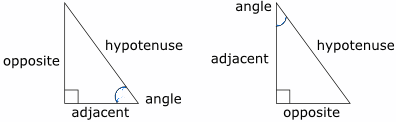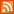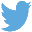Bucaro TecHelpHTTPS Encryption not required because no account numbers orpersonal information is ever requested or accepted by this siteCustom Search# Java Script Math.sin Method

Sine is a trigonometric function. Based on a right triangle. It's the ratio of the length of the opposite side to the length of the hypotenuse.

sine A = opposite/hypotenuseIn identifying the sides of a right triangle, the side next to the angle for which we are using the trigonometric function is called the adjacent side. The side opposite that angle is called the opposite side.

If you know an angle and the length of the side opposite the angle, you can use the sine of the angle to calculate the length of the hypotenuse.

length of hypotenuse = length of opposite/sine of angle

Example

```angle = 40 degrees
opposite side = 9
```

Java Script Using Math.sin Method

```<script type="text/javascript">

var angle = 40;
var opposite = 9;

var hypotenuse = opposite/Math.sin(40 * Math.PI/180);

 .menubtn { margin-top:10px; margin-left:50px; width:220px; opacity:1; border-style:solid; border-color:#009000; background-color:#66ff66; transition-property: opacity; transition-duration: 1s; transition-timing-function: ease-out; } .menubtn:hover { opacity:1; background-color:#e5ff23; } Menu - More Java Script Quick ReferenceRSS FeedFollow @Stephen Bucaro